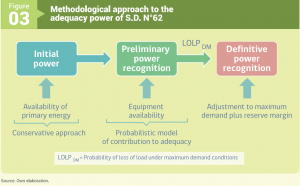Menú Principal

# Annex 3: Adequacy power (methodology S.D. N°62)

The objective of the method of calculating of the adequacy power is to distribute the annual revenues from the sale of capacity to the customers of a system among the owners of the generating plants and to determine the power transfers between companies. This is determined from the adequacy power and the existing peak demand commitments. In this way, each owner of generation means must be able to satisfy his own commitments for peak demand considering his adequacy power and that acquired to other companies.

Each generating unit is assigned an adequacy power depending on the uncertainty associated with the availability of the main input of generation that is used and the unavailability of the unit and the installations that interconnect it, characterized by the initial power and the preliminary adequacy power, respectively. The general scheme of the procedure of calculation of adequacy power that establishes the S.D. N°62 is illustrated in Figure 3.The following describes each of the stages and concepts used in the calculation process:

Initial Power of generating units whose source is non-conventional
For units such as geothermal, wind, solar, biomass, tidal, cogeneration and small hydropower (up to 20 MW), the initial power will be determined in a way equivalent to conventional, depending on the resource you use. For this, it is considered the worst scenario of average annual availability of main input that corresponds. The information must be given to the Coordinator by each owner. If sufficient statistical information is not available, use available information by zone or region in which the unit is located.

Initial Power of hydroelectric plants
For hydroelectric plants, the statistics of affluent flows corresponding to the average of the two hydrological years of lower energy of the available statistics are used. To do this, the regulation (S.D. N°62 of 2006) classifies the hydroelectric plants according to their capacity of regulation in: central with capacity of daily regulation or superior, central with capacity of regulation intraday and central without capacity of regulation . It also distinguishes hydroelectric plants in series:

• Generating units belonging to plants with daily or higher regulation capacity are considered an initial energy equal to the average energy stored at 1 April, during the last 20 years, including the year of calculation.
• Generating units with intraday regulation capacity are considered as regulating capacity but are not considered the initial energy.
• The initial power of the units without regulation capacity is determined according to the equivalent power to the average annual affluent caudal of the 2 hydrological conditions of lower affluent energy.

The calculation methodology for power plants with control capacity is performed by means of a procedure that seeks to distribute the total hydraulic power of the system following a filling of the annual load duration curve. This, respecting the models of the watersheds. The initial joint power (regulation power) obtained at the time of greatest demand is assigned to the plants with regulatory capacity prorate of the annual power of regulation provided to the system.

Thermal Initial Power
It is determined based on the lowest average annual availability observed for the main input for the last 5 years prior to the year of calculation. If the plant demonstrates operating capacity with an alternative input, then the adequacy power will be calculated as an equivalent generating unit based on the operating characteristics of each unit with the main and alternative input.

For the calculation of the preliminary adequacy power, the probabilistic model determined by the Coordinator is used, which must consider for each generating unit its initial power, unavailability, maintenance period and own consumptions.

In its first part, the initial power is reduced by the following factors that account for its annual availability:

• Own consumption
• Scheduled maintenance
• Failure rates (IFOR) and deteriorated states

The capacity resulting from these reductions are introduced into a probabilistic model that considers “the contribution to adequacy system” of each power plant to determine its preliminary adequacy power.

Definitive Adequacy Power and Theoretical Reserve Margin (MRT)
In this part of the calculation process, the preliminary power of each generator is scaled by a factor – the same for each one – so that the sum of definitive power is equal to the peak demand of the system.

On the other hand, the basic price of capacity, determined by the CNE, incorporates a scaling given by the Theoretical Reserve Margin (MRT) of the system. The MRT corresponds to the minimum over-equipment in generation capacity that allows supplying the tip power in a system with a determined adequacy. This margin is calculated from the Power Margin (MP), equal to the total Initial Power of the system, using a linear function whose result is bounded lower by a minimum MRT of 10%. The function acts in an inverse way so that at higher MP a lower MRT is obtained and vice versa. For usual MP values the MRT will move in a range of 10% to 15%.